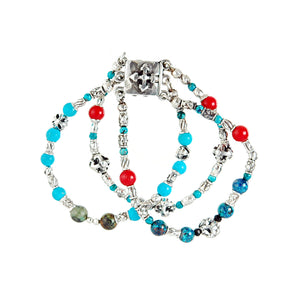Liquid error (image-logic line 10): divided by 0Liquid error (image-logic line 10): divided by 0
Liquid error (image-logic line 10): divided by 0
Liquid error (image-logic line 10): divided by 0